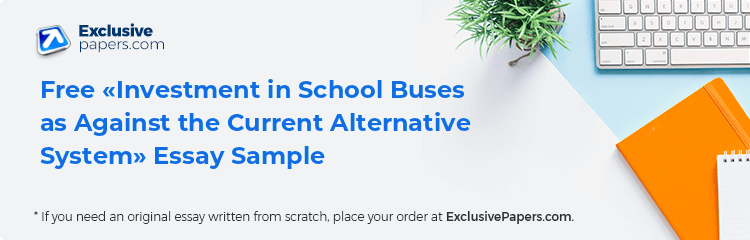This paper presents a discussion regarding capital investments. The aim is to understand the concepts behind decision making concerning any capital investments. The revenues to be earned and the expenses incurred constitute important inputs towards an informed and appropriate decision being drawn. In this case, a private school is considering whether to invest in its own school buses or to continue with the services it has since outsourced or contracted. The accruing costs and revenues have been given which are in turn computed to get the Payback Period, the Accounting Rate of Return and the Net Present Value. The alternative option, currently being used, has also been given with its values for consideration.

In the first part, a table has been generated showing the net cash flows that would arise out of investing in the school buses. The table is here below presented;

 year 0 1 2 3 4 5 Revenue 0 300,000 330,000 350,000 380,000 400,000 Total costs 600,000 171,000 183,000 191,000 190,000 200,000 Net cash flow -600,000 129,000 147,000 159,000 190,000 200,000

2. (i) Calculation of the Payback Period. This is the determination of the length in time it takes to recover the initial cost of an investment. It does not take into account the time value of money concept. It also disregards the total stream of the cash flows since it is concerned with the period up which the initial investment cost is recovered.

•

0

Preparing Orders

•

0

Active Writers

•

0%

Positive Feedback

•

0

Support Agents

• Total price

It however provides an easy way to analyze a project without many complexities. It is calculated as shown below;

The initial investment cost is 600,000, earnings are shown in the table above. The totals are; 129,000+147,000 = 276,000+159,000 = 435,000+190,000 = 625,000, this has surpassed the initial cost of the investment. So the period is 3 years and the months calculated as below.

600,000-435,000 = 165,000/190,000 = 0.8684(12 months) =10.42 months

The Payback Period is therefore 3 years and 10 months.

(ii) Calculation of the Average Rate of Return. This is used to evaluate investment opportunities by comparing the accounting rates of return with a required rate of return. It is calculated by dividing the Estimated Average Profits by the Estimated Average Investment. It is computed as shown below.

 year 0 1 2 3 4 5 Cash inflow 0 300,000 330,000 350,000 380,000 400,000 Total expenses 600,000 171,000 183,000 191,000 190,000 200,000 Depreciation 120,000 120,000 120,000 120,000 120,000 Accounting income 9,000 27,000 39,000 70,000 80,000

Total accounting income is 225,000. The average is 225,000/5 which equals to 45,000. The average investment is 600,000/2 which is 300,000. This is so because the investment has no salvage value at the end of its lifetime. So the Average Rate of Return is arrived at as follows;

45,000/300,000 = 0.15, which is 15%.

(iii) The last calculation is the Net Present Value of the project. This is the net worthiness of the investment taking into consideration the concept of time value of money, the amounts of revenues and costs accruing to the investment. It is calculated by discounting the future cash flows into present sums. In this case, the net cash flows are discounted so that present sums are then summed up to arrive at the present values. It is computed as shown below:

1st year, Net cash flow, 129,000/1.12 = 115,179

2nd Year, Net Cash flow, 147,000/1.122 = 117,188

3rd Year, Net Cash flow, 159,000/1.123 = 113,173

4th Year, Net Cash flow, 190,000/1.124 = 120,748

5th Year, Net Cash flow, 200,000/1.125 = 113,485

Total present values = 579,773

Net Present Value of the project would be, the net incomes less the initial capital outlay. This is 579,773 less 600,000 which is -20,227.

3. A Memo is to be written to the Investment Manager explaining whether to go for the school buses as an investment or to continue with the current system. The current alternative has its values as; Payback Period is 3 years, ARR is 30% and NPV is 140,000. Here below is the memo.

Toll free

Memo

Re: Investment in school buses as against the current alternative system

Based on the calculations above, investing in the school buses would be inappropriate. This is because in the first instance, it has a negative Present Value. This means that the investment does not make any return to the company. The present values of the total costs exceed the present value of the total revenues. This option should therefore not be pursued since it leads to losses. The current alternative being used should instead continue. It has a positive Present Value of 140,000, meaning that the company earns or saves that amount by utilizing the means. Its Payback Period is also shorter than the one of investing in the buses. It is 3 years against the other of 3 years and 10 months. The ARR of the current system is also higher than the alternative of buying the buses. It is at 30% against 15% in the new investment. Therefore it is only prudent from the above facts that the investment be ignored since the present means are better than investing in the new ones by buying own buses.

Silver member

5% OFF

Gold member

10% OFF

Platinum member

15% OFF

What Our Customers Say